## Recommended Posts

Hello everyone, please visit my temporary site : http://secondbook.onlinewebshop.net/ please try to buy product more than 3 , at least 4 product. I don't know how to say it because I don't know the name, when you buy product it will shown number "1" on Order page column. But if you buy product more than 3 , the product still keep shown into bellow and page number not added on order page column. Q : How to make it , so that it will be shown "2" on Order page column and the product that ordered displayed at page 2 , if product that ordered are more than 3? Note : this is using session not using database to display it. Q : what the name of this number link? this is my code : this code to display the navigation number link.

`<?php\$total_order = count(\$_SESSION['cart']);if(!\$_SESSION['m_user']){\$op = 1;for(\$w=0;\$w<\$total_order;\$w+=3){if(\$total_order>3){  \$num_link = "<div class=\"cart_num\"><a href=\"?p=basket&o=\$w&ot=\$total_order\">\$op</a></div>";}else{  \$num_link = "<div class=\"cart_num\"><a href=\"?p=basket&o=0&ot=\$total_order\">1</a></div>";}}echo"<div class=\"pr_page\" style=\"border-bottom:1px solid black;\">Order Page </div>	   <div class=\"pr_number\" style=\"border-bottom:1px solid black;float:left;width:472px\">	 \$num_link	   </div>";	\$op++;}?>`

this code to display order

`<?\$qty		= \$_POST[qty];//if there is an product_id and that product_id doesn't exist display an error messageif(\$product_id && !productExists(\$product_id)) {  die("Error. Product Doesn't Exist");}switch(\$action) { //decide what to do   case "add":   \$_SESSION['cart'][\$product_id]++;  break;   case "remove":unset(\$_SESSION['cart'][\$product_id]);  break;   case "empty":   unset(\$_SESSION['cart']);  break;   case "change":	\$_SESSION['cart'][\$product_id]=+\$qty;	if(preg_match("/[.]/",\$qty)){\$_SESSION['cart'][\$product_id]=+1;}	if(\$_SESSION['cart'][\$product_id] == 0){unset(\$_SESSION['cart'][\$product_id]);}  break; }if(\$_SESSION['cart']&&!\$_SESSION['m_user']) {				\$total_order = count(\$_SESSION['cart']);	echo"<div class=\"b_title\">   <div align=\"center\"><b>Your total order on basket : <font color=\"#000066\">\$total_order</font></b></div>   </div>";      foreach(\$_SESSION['cart'] as \$product_id => \$quantity) {	\$sql = sprintf("SELECT p_id, p_title, p_price, p_image FROM product WHERE p_id = %d;",		\$product_id);		\$result = mysql_query(\$sql);		//Only display the row if there is a product (though there should always be as we have already checked)	if(mysql_num_rows(\$result) > 0) {  	 list(\$p_id, \$p_title, \$p_price, \$p_image) = mysql_fetch_row(\$result);  	 \$line_cost = \$p_price * \$quantity;  //work out the line cost	 \$total = \$total + \$line_cost;   //add to the total cost 	   echo"	<div class=\"b_content\">		 <form method=\"post\" action=\"?p=basket&action=change&id=\$product_id\">		  <div class=\"b_img\"><img src=\"product/\$p_image\" width=\"105px\" height=\"152px\" border=\"1\"/></div>	   <div class=\"b_detail\">		   <div class=\"b_columnL\">Book Name : </div> <div class=\"b_columnR\"><b>\$p_title</b></div>		   <div class=\"b_columnL\">Price Per Book : </div> <div class=\"b_columnR\">\$p_price</div>		   <div class=\"b_columnL\">Total Item : </div> <div class=\"b_columnR\"><input type=\"text\" name=\"qty\" style=\"width:20px;text-align:center;border:1px solid #000066;\" maxlength=\"3\" value=\"\$quantity\" /></div>		   <div class=\"b_columnL\">Total Price : </div> <div class=\"b_columnR\">USD \$line_cost</div>		   <div class=\"b_columnL\"><a href=\"basket.php?action=remove&id=\$product_id\">Delete</a></div> <div class=\"b_columnR\"><input type=\"submit\" value=\"RECALCULATE\" /></div>				</div>	  </form>	  </div>";	}	}     \$checkout ="<div align=\"left\" style=\"float:left\"><a href=\"?p=basket\">Back to shop</a></div><div align=\"right\" style=\"float:right\"><a href=\"?p=pass\">Checkout</a></div>";   echo "<div class=\"checkout\" style=\"float:left;padding-left:24px;\"><a href=\"basket.php?action=empty\">Empty Order</a></div> <div class=\"checkout\">Total that must be pay : USD <b>\$total</b> <hr size=\"1\" color=\"#000000\" />\$checkout</div>";}else if(!\$_SESSION['m_user']){echo "<div class=\"b_title\"><b>You have no item in your basket cart</b></div>";}else{include("application-basket.php");}//function to check if a product existsfunction productExists(\$product_id) {   //use sprintf to make sure that \$product_id is inserted into the query as a number - to prevent SQL injection   \$sql = sprintf("SELECT * FROM product WHERE p_id = %d;",	   \$product_id);     return mysql_num_rows(mysql_query(\$sql)) > 0;}?>`

##### Share on other sites

I don't really understand what you're saying the problem is or what your questions are, but the code doesn't look right. I don't understand what you're trying to do here:

`for(\$w=0;\$w<\$total_order;\$w+=3){if(\$total_order>3){\$num_link = "<div class=\"cart_num\"><a href=\"?p=basket&o=\$w&ot=\$total_order\">\$op</a></div>";}else{\$num_link = "<div class=\"cart_num\"><a href=\"?p=basket&o=0&ot=\$total_order\">1</a></div>";}}`

What is the point of that code? Why do you have the loop there if you're just going to print a single link? Why are you incrementing \$w by 3? Why are you checking if \$total_order is greater than 3? I don't understand what you're trying to do with that code. For these 2 lines: \$_SESSION['cart'][\$product_id]=+\$qty;if(preg_match("/[.]/",\$qty)){\$_SESSION['cart'][\$product_id]=+1;} "=+" is the same as "=". It's the same as this: \$_SESSION['cart'][\$product_id] = (+\$qty); If you want to add those values then you use +=, not =+. That regular expression doesn't make a lot of sense either, you're checking if \$qty contains any characters at all. It's the same as this: if (\$qty != '')

##### Share on other sites

I don't really understand what you're saying the problem is or what your questions are, but the code doesn't look right. I don't understand what you're trying to do here:
Probably I need to attach images. This is image on normal on my temporary site (http://secondbook.onlinewebshop.net/), and this occurs if user buy product as much as 3 : Image 1 :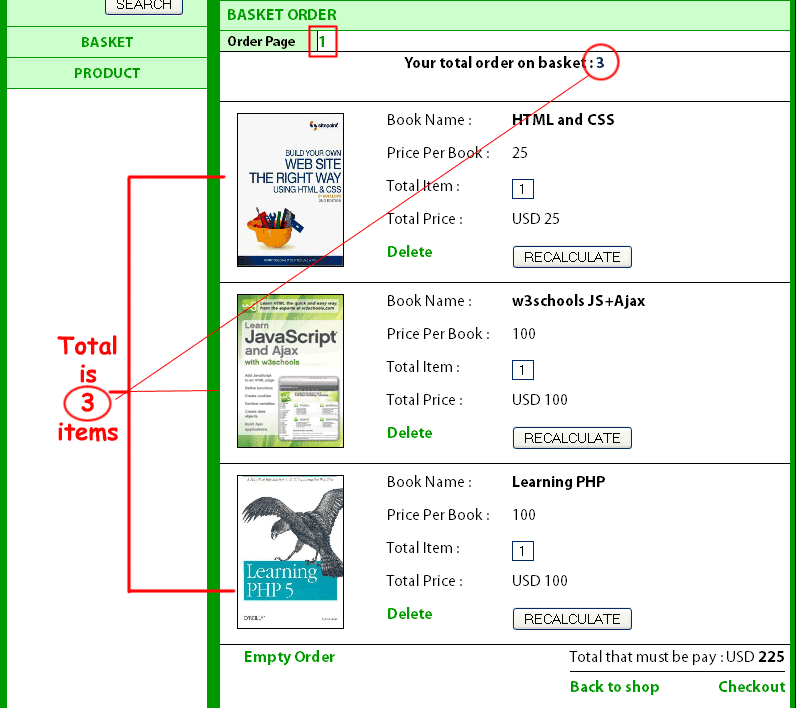and this is my problem / question : Image 2 :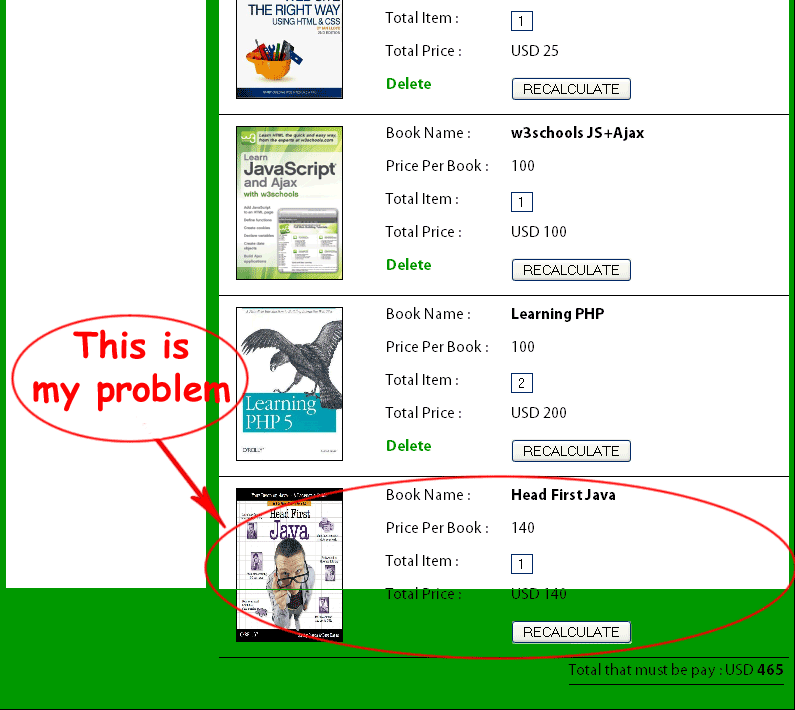The next order displayed on same page (1), and this occurs if user buy product more than 3. Q : How to make it so that it shown like this : Image 3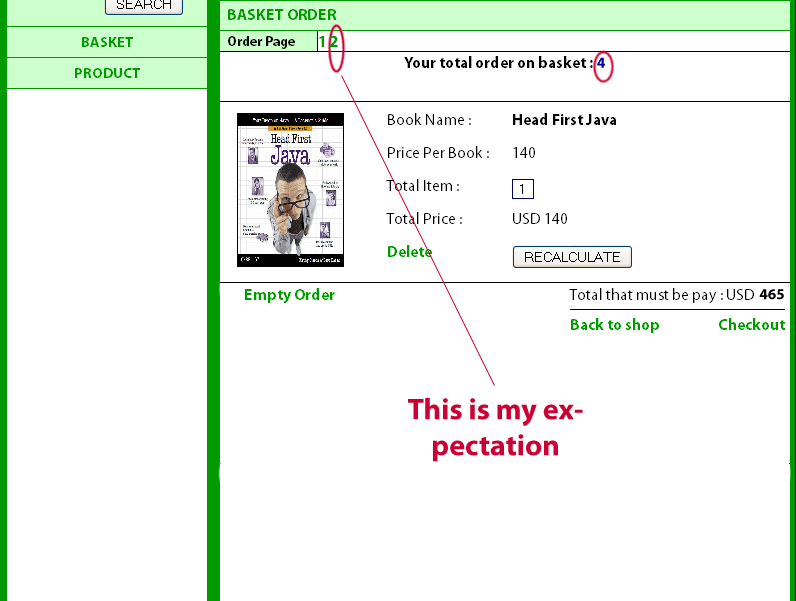My point / target is : create navigation link page, so that , if user buy product more than 3 , link 2 appear on Order Page (like image 3 on above). And the next product will be shown on page 2, and if user click link number 1 it's back to previous page (like image 1 on above) and page 2 not disappear and otherwise.In short, after page contains 3 product., the next link navigation appear (regularly). My code that contains loop function , purpose to create link navigation (2) or more (based on total order). And this is my problem. please help me Thanks
##### Share on other sites

If you do a search for pagination you'll find examples for that. You need to know how many items to display on the page and which page to display and you can jump to the appropriate starting point in the array and display the items for that page. To calculate how many pages you have, and how many page links you need to show, you just divide the total number of items by the number per page.

##### Share on other sites

I had try but it's not successful and this is the result : Image I :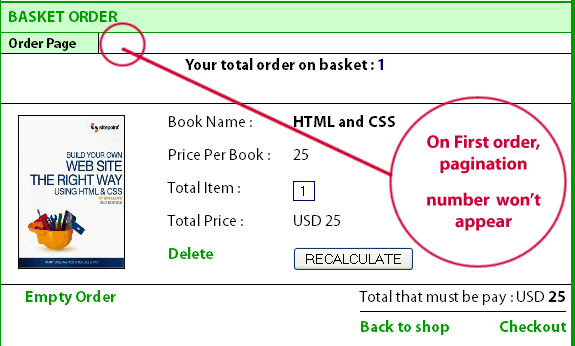and the problem still same : Image 2 :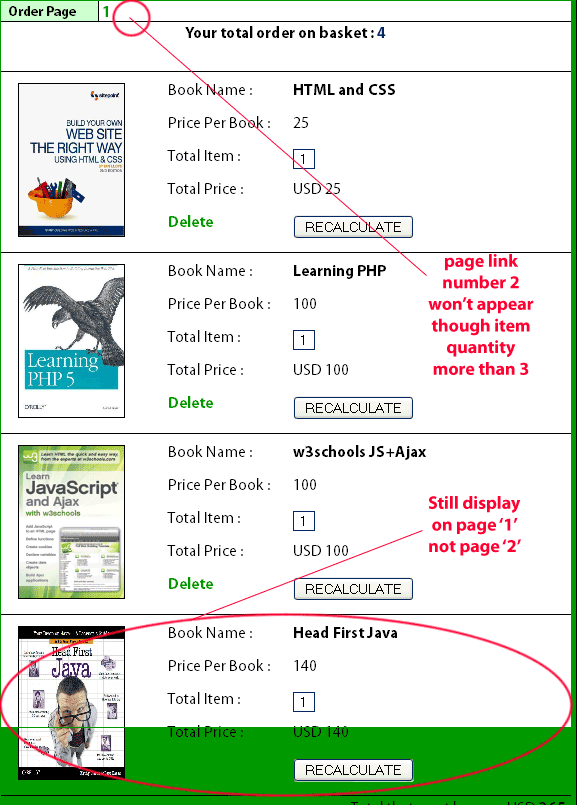And I don't know why this is occurred : Image 3 :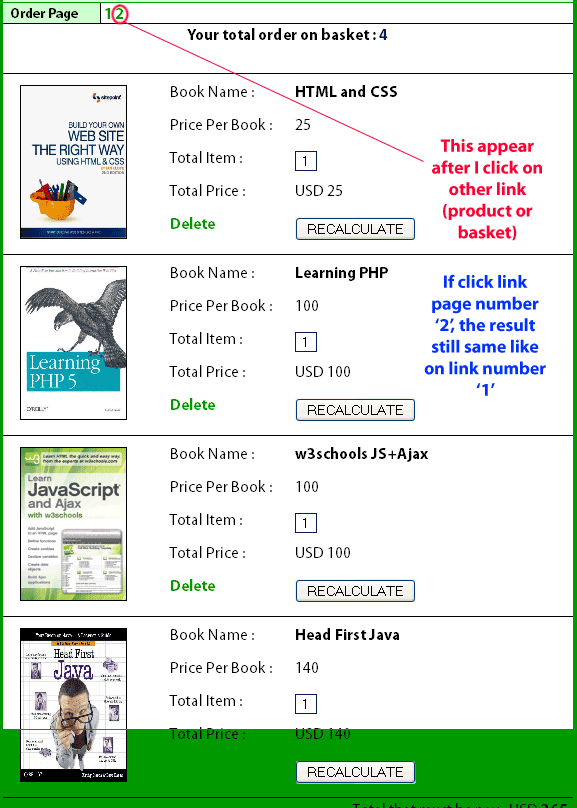This is my code :

`<?php\$product_id = \$_GET[id];  //the product id from the URL\$action  = \$_GET[action]; //the action from the URL?><div class="title">BASKET ORDER</div><div class="pr_page" style="border-bottom:1px solid black;">Order Page </div>   <div class="pr_number" style="border-bottom:1px solid black;float:left;width:472px">   <?	   \$total_order = count(\$_SESSION['cart']);	   if(!\$_SESSION['m_user']){  \$op = 1;  for(\$w=0;\$w<\$total_order;\$w+=3){   if(\$total_order>3){	echo"<div class=\"cart_num\"><a href=\"?p=basket&o=\$w&ot=\$total_order\">\$op</a></div>";	\$op++;   }else{	echo"<div class=\"cart_num\"><a href=\"?p=basket&o=0&ot=\$total_order\">1</a></div>";	}   }  }   \$x=\$o+3;  if(\$o==""||\$o==0||preg_match("/[a-z]/",\$o)){		 \$o=0;		}  if(\$total_order<=3){  \$y=\$total_order;  }else{  \$y=\$x;  }  ?>   </div><?\$qty		= \$_POST[qty];//if there is an product_id and that product_id doesn't exist display an error messageif(\$product_id && !productExists(\$product_id)) {  die("Error. Product Doesn't Exist");}switch(\$action) { //decide what to do   case "add":   \$_SESSION['cart'][\$product_id]++; //add one to the quantity of the product with id \$product_id  break;   case "remove":   unset(\$_SESSION['cart'][\$product_id]);  break;   case "empty":   unset(\$_SESSION['cart']); //unset the whole cart, i.e. empty the cart.  break;   case "change":	\$_SESSION['cart'][\$product_id]=+\$qty;	if(preg_match("/[.]/",\$qty)){\$_SESSION['cart'][\$product_id]=+1;}	if(\$_SESSION['cart'][\$product_id] == 0){unset(\$_SESSION['cart'][\$product_id]);}  break; }if(\$_SESSION['cart']&&!\$_SESSION['m_user']) {				\$total_order = count(\$_SESSION['cart']);	echo"<div class=\"b_title\">   <div align=\"center\"><b>Your total order on basket : <font color=\"#000066\">\$total_order</font></b></div>   </div>";      foreach(\$_SESSION['cart'] as \$product_id => \$quantity) {  	//get the name, description and price from the database - this will depend on your database implementation.	//use sprintf to make sure that \$product_id is inserted into the query as a number - to prevent SQL injection	\$sql = sprintf("SELECT p_id, p_title, p_price, p_image FROM product WHERE p_id = %d;",		\$product_id);		\$result = mysql_query(\$sql);		//Only display the row if there is a product (though there should always be as we have already checked)	if(mysql_num_rows(\$result) > 0) {  	 list(\$p_id, \$p_title, \$p_price, \$p_image) = mysql_fetch_row(\$result);  	 \$line_cost = \$p_price * \$quantity;  //work out the line cost	 \$total = \$total + \$line_cost;   //add to the total cost 	   echo"	<div class=\"b_content\">		 <form method=\"post\" action=\"?p=basket&action=change&id=\$product_id\">		  <div class=\"b_img\"><img src=\"product/\$p_image\" width=\"105px\" height=\"152px\" border=\"1\"/></div>	   <div class=\"b_detail\">		   <div class=\"b_columnL\">Book Name : </div> <div class=\"b_columnR\"><b>\$p_title</b></div>		   <div class=\"b_columnL\">Price Per Book : </div> <div class=\"b_columnR\">\$p_price</div>		   <div class=\"b_columnL\">Total Item : </div> <div class=\"b_columnR\"><input type=\"text\" name=\"qty\" style=\"width:20px;text-align:center;border:1px solid #000066;\" maxlength=\"3\" value=\"\$quantity\" /></div>		   <div class=\"b_columnL\">Total Price : </div> <div class=\"b_columnR\">USD \$line_cost</div>		   <div class=\"b_columnL\"><a href=\"basket.php?action=remove&id=\$product_id\">Delete</a></div> <div class=\"b_columnR\"><input type=\"submit\" value=\"RECALCULATE\" /></div>				</div>	  </form>	  </div>";	}	}     //show the total   \$checkout ="<div align=\"left\" style=\"float:left\"><a href=\"?p=basket\">Back to shop</a></div><div align=\"right\" style=\"float:right\"><a href=\"?p=pass\">Checkout</a></div>";   echo "<div class=\"checkout\" style=\"float:left;padding-left:24px;\"><a href=\"basket.php?action=empty\">Empty Order</a></div> <div class=\"checkout\">Total that must be pay : USD <b>\$total</b> <hr size=\"1\" color=\"#000000\" />\$checkout</div>";}else if(!\$_SESSION['m_user']){echo "<div class=\"b_title\" style=\"color:#000099\"><b>You have no item in your basket cart</b></div>";}else{include("application-basket.php");}//function to check if a product existsfunction productExists(\$product_id) {   //use sprintf to make sure that \$product_id is inserted into the query as a number - to prevent SQL injection   \$sql = sprintf("SELECT * FROM product WHERE p_id = %d;",	   \$product_id);     return mysql_num_rows(mysql_query(\$sql)) > 0;}?>`

Q : What's mistake on my code? Note : pagination tutorial that I found on google using database to display their data, I still didn't found if using SESSION. please help me Thanks

##### Share on other sites

Note : pagination tutorial that I found on google using database to display their data, I still didn't found if using SESSION.
It doesn't matter where the data comes from. That's not the point. The point is how to split up a list of items across pages, it doesn't matter if you get the data from a database, or a session, or a file, or XML, or someone typing something in. That's not the important part. If you find one description that only describes one way and you can't figure out how to adapt it to your situation then look for another one. You need to figure out how many page links to show. That means you need to know how many items you have, how many items you want to show per page, and you divide to get the number of pages to show links for. You're not doing that, you have a weird for loop that I asked you questions about that you didn't answer. I don't know what you're trying to do there, but all you need to do is calculate how many pages are needed to show the order, then loop that many times and print the link for each page. The only thing the URL needs is a page number, e.g. cart.php?page=1, cart.php?page=2, etc. It's just a basic loop, all you need to do is divide the number of items in the cart by the number of items to show on each page, round that number up, and loop that many times. That's it. In order to show the correct set of items for a given page then you get the page number from the URL, default that to 1 if it's not in the URL, subtract 1 from the page number and multiply by the number of items per page, and the resulting number is the index that you start looping at in the array of items. That's all there is to it. One small piece of code to do the loop to print the page numbers, another small piece of code to figure out where to start looping through the array of items to show.
##### Share on other sites

Before I continue my post I need to clarification :

you have a weird for loop that I asked you questions about that you didn't answer. I don't know what you're trying to do there
I think I had give you answer on post 3 , please see : My code that contains loop function , purpose to create link navigation (2) or more (based on total order). And this is my problem. So, I create for loop function is for to create pagination.______________________________________________________________________________________________________________________ Today I found tutorial about pagination from : http://papermashup.com/demos/php-pagination/ I try it's work but there is some part that become my problem : CODE I :
`<?phpinclude('connect.php');[font="helvetica, arial, sans-serif"]\$tableName="p_order";\$targetpage = "index.php";\$limit = 2; \$query = "SELECT COUNT(*) as num FROM \$tableName";\$total_pages = mysql_fetch_array(mysql_query(\$query));\$total_pages = \$total_pages[num]; \$stages = 3;\$page = mysql_real_escape_string(\$_GET['page']);if(\$page){  \$start = (\$page - 1) * \$limit;}else{  \$start = 0;  }`

CODE II :

`// Get page data\$query1 = "SELECT * FROM \$tableName LIMIT \$start, \$limit";\$result = mysql_query(\$query1); // Initial page num setupif (\$page == 0){\$page = 1;}\$prev = \$page - 1;\$next = \$page + 1;	  \$lastpage = ceil(\$total_pages/\$limit);\$LastPagem1 = \$lastpage - 1;	  \$paginate = '';`

CODE III :

`if(\$lastpage > 1){   \$paginate .= "<div class='paginate'>";  // Previous  if (\$page > 1){   \$paginate.= "<a href='\$targetpage?page=\$prev'>previous</a>";  }else{   \$paginate.= "<span class='disabled'>previous</span>"; }    // Pages  if (\$lastpage < 7 + (\$stages * 2)) // Not enough pages to breaking it up  {   for (\$counter = 1; \$counter <= \$lastpage; \$counter++)   {	if (\$counter == \$page){	 \$paginate.= "<span class='current'>\$counter</span>";	}else{	 \$paginate.= "<a href='\$targetpage?page=\$counter'>\$counter</a>";}	   }  }  elseif(\$lastpage > 5 + (\$stages * 2)) // Enough pages to hide a few?  {   // Beginning only hide later pages   if(\$page < 1 + (\$stages * 2))   {	for (\$counter = 1; \$counter < 4 + (\$stages * 2); \$counter++)	{	 if (\$counter == \$page){	  \$paginate.= "<span class='current'>\$counter</span>";	 }else{	  \$paginate.= "<a href='\$targetpage?page=\$counter'>\$counter</a>";}		}	\$paginate.= "...";	\$paginate.= "<a href='\$targetpage?page=\$LastPagem1'>\$LastPagem1</a>";	\$paginate.= "<a href='\$targetpage?page=\$lastpage'>\$lastpage</a>";   }   // Middle hide some front and some back   elseif(\$lastpage - (\$stages * 2) > \$page && \$page > (\$stages * 2))   {	\$paginate.= "<a href='\$targetpage?page=1'>1</a>";	\$paginate.= "<a href='\$targetpage?page=2'>2</a>";	\$paginate.= "...";	for (\$counter = \$page - \$stages; \$counter <= \$page + \$stages; \$counter++)	{	 if (\$counter == \$page){	  \$paginate.= "<span class='current'>\$counter</span>";	 }else{	  \$paginate.= "<a href='\$targetpage?page=\$counter'>\$counter</a>";}		}	\$paginate.= "...";	\$paginate.= "<a href='\$targetpage?page=\$LastPagem1'>\$LastPagem1</a>";	\$paginate.= "<a href='\$targetpage?page=\$lastpage'>\$lastpage</a>";   }   // End only hide early pages   else   {	\$paginate.= "<a href='\$targetpage?page=1'>1</a>";	\$paginate.= "<a href='\$targetpage?page=2'>2</a>";	\$paginate.= "...";	for (\$counter = \$lastpage - (2 + (\$stages * 2)); \$counter <= \$lastpage; \$counter++)	{	 if (\$counter == \$page){	  \$paginate.= "<span class='current'>\$counter</span>";	 }else{	  \$paginate.= "<a href='\$targetpage?page=\$counter'>\$counter</a>";}		}   }  }		// Next  if (\$page < \$counter - 1){   \$paginate.= "<a href='\$targetpage?page=\$next'>next</a>";  }else{   \$paginate.= "<span class='disabled'>next</span>";   }    \$paginate.= "</div>";  }echo \$total_pages.' Results';// paginationecho \$paginate;?>`

CODE IV :

`<?php   while(\$row = mysql_fetch_array(\$result))  {   echo '<li>'.\$row['o_title'].'</li>';   } 	?>`

I understand the mean of this code, Code I : contains database that have data to display as total,Code II : contains database that have data to display on browser and it using limit (on sql taq) and \$limit to control how many item to show per page,Code III : Code to display pagination number , like this picture :Code IV : code to display item / contents, like this image. Note : Code on above deliberately I divided,so that it's not painful to be read My problem is : All code and tutorial on above using database to make limit , of course it's using mysql_query("SELECT * FROM \$tableName LIMIT \$start, \$limit"). How to make it ,so that if I using session the data from session can be displayed appropriate with limit that determined and can be combined with my session code on below? In short how to divide data from session My code to display session order is like this :

`if(\$_SESSION['cart']&&!\$_SESSION['m_user']) {				\$total_order = count(\$_SESSION['cart']);	echo"<div class=\"b_title\">   <div align=\"center\"><b>Your total order on basket : <font color=\"#000066\">\$total_order</font></b></div>   </div>";      foreach(\$_SESSION['cart'] as \$product_id => \$quantity) {  	//get the name, description and price from the database - this will depend on your database implementation.	//use sprintf to make sure that \$product_id is inserted into the query as a number - to prevent SQL injection	\$sql = sprintf("SELECT p_id, p_title, p_price, p_image FROM product WHERE p_id = %d;",		\$product_id);		\$result = mysql_query(\$sql);		//Only display the row if there is a product (though there should always be as we have already checked)	if(mysql_num_rows(\$result) > 0) {  	 list(\$p_id, \$p_title, \$p_price, \$p_image) = mysql_fetch_row(\$result);  	 \$line_cost = \$p_price * \$quantity;  //work out the line cost	 \$total = \$total + \$line_cost;   //add to the total cost 	   echo"	<div class=\"b_content\">		 <form method=\"post\" action=\"?p=basket&action=change&id=\$product_id\">		  <div class=\"b_img\"><img src=\"product/\$p_image\" width=\"105px\" height=\"152px\" border=\"1\"/></div>	   <div class=\"b_detail\">		   <div class=\"b_columnL\">Book Name : </div> <div class=\"b_columnR\"><b>\$p_title</b></div>		   <div class=\"b_columnL\">Price Per Book : </div> <div class=\"b_columnR\">\$p_price</div>		   <div class=\"b_columnL\">Total Item : </div> <div class=\"b_columnR\"><input type=\"text\" name=\"qty\" style=\"width:20px;text-align:center;border:1px solid #000066;\" maxlength=\"3\" value=\"\$quantity\" /></div>		   <div class=\"b_columnL\">Total Price : </div> <div class=\"b_columnR\">USD \$line_cost</div>		   <div class=\"b_columnL\"><a href=\"basket.php?action=remove&id=\$product_id\">Delete</a></div> <div class=\"b_columnR\"><input type=\"submit\" value=\"RECALCULATE\" /></div>				</div>	  </form>	  </div>";	}	}     //show the total   \$checkout ="<div align=\"left\" style=\"float:left\"><a href=\"?p=basket\">Back to shop</a></div><div align=\"right\" style=\"float:right\"><a href=\"?p=pass\">Checkout</a></div>";   echo "<div class=\"checkout\" style=\"float:left;padding-left:24px;\"><a href=\"basket.php?action=empty\">Empty Order</a></div> <div class=\"checkout\">Total that must be pay : USD <b>\$total</b> <hr size=\"1\" color=\"#000000\" />\$checkout</div>";}else if(!\$_SESSION['m_user']){echo "<div class=\"b_title\" style=\"color:#000099\"><b>You have no item in your basket cart</b></div>";}else{include("application-basket.php");}`

##### Share on other sites

instead of looping through a bunch of DB results, you just have to loop through your cart in instead. You have to programmatically make the start and end points, which is very simple in practice.

`\$ITEMS_PER_PAGE = 3; \$page = \$_GET['page'];  //for our example. it is equal to 2\$start = (\$page - 1) * \$ITEMS_PER_PAGE;  //would be 3\$end = \$start + (\$ITEMS_PER_PAGE - 1);   //would be 5 for(\$i = \$start; \$i <= \$end; \$i++){  if(\$_SESSION['cart'][\$i]){	 //show this item on the page  }}`

using that as a setup, you can can start calculating other things like next and previous, or other pages. It might help to write it out, or just echo values out on the page before actually using the data. Keep it simple and slowly build up the functionality. Just work on aspect of it at a time.

Edited by thescientist
##### Share on other sites

Code 1 figures out how many items to display and defines variables like the number to show per page and the item to start with. Code 2 gets the data and defines more variables, like the page numbers of the next and previous pages to use for the next and previous buttons. It also calculates how many pages there are in total. Code 3 uses the variables that were previously defined to create the buttons and page links, and code 4 displays the data. Notice I didn't use the word "database" in that explanation. Code 3 in particular doesn't do a single thing with the database in order to print the links. The only thing they use the database for are to figure out how many items there are (you already know that, because they're sitting in an array), and to get the actual data (you already have the data). I already told you what you need to do:

You need to figure out how many page links to show. That means you need to know how many items you have, how many items you want to show per page, and you divide to get the number of pages to show links for. You're not doing that, you have a weird for loop that I asked you questions about that you didn't answer. I don't know what you're trying to do there, but all you need to do is calculate how many pages are needed to show the order, then loop that many times and print the link for each page. The only thing the URL needs is a page number, e.g. cart.php?page=1, cart.php?page=2, etc. It's just a basic loop, all you need to do is divide the number of items in the cart by the number of items to show on each page, round that number up, and loop that many times. That's it. In order to show the correct set of items for a given page then you get the page number from the URL, default that to 1 if it's not in the URL, subtract 1 from the page number and multiply by the number of items per page, and the resulting number is the index that you start looping at in the array of items. That's all there is to it. One small piece of code to do the loop to print the page numbers, another small piece of code to figure out where to start looping through the array of items to show.
That's what you need to do, you need to calculate or define those values and you can basically use the exact same code in code 3 to print the list of links. Instead of getting the data from a database you already have it in an array, you just need to figure out where to start in the array and how many to show.
##### Share on other sites

Hi , Today I had try to combine code and this is the result :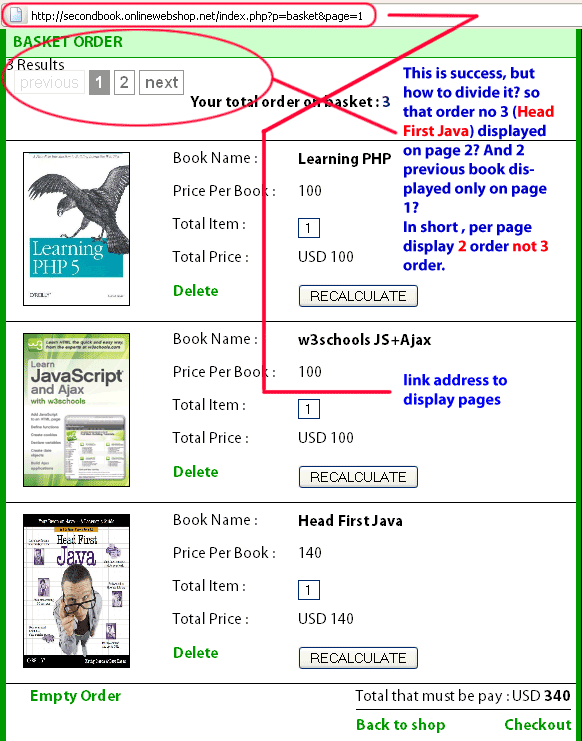And these the code : Code 1 :

`<?\$page = mysql_real_escape_string(\$_GET['page']);\$total_pages = count(\$_SESSION['cart']);\$limit = 2;if(\$page){  \$start = (\$page - 1) * \$limit;}else{  \$start = 0;  } if (\$page == 0){\$page = 1;}\$prev = \$page - 1;\$next = \$page + 1;	  \$lastpage = ceil(\$total_pages/\$limit);\$LastPagem1 = \$lastpage - 1;	 \$paginate = '';`

Code 2 :

`if(\$lastpage > 1){  \$paginate .= "<div class='paginate'>";  // Previous  if (\$page > 1){   \$paginate.= "<a href='?p=basket&page=\$prev'>previous</a>";  }else{   \$paginate.= "<span class='disabled'>previous</span>"; }     // Pages  if (\$lastpage < 7 + (\$stages * 2)) // Not enough pages to breaking it up  {   for (\$counter = 1; \$counter <= \$lastpage; \$counter++)   {	if (\$counter == \$page){	 \$paginate.= "<span class='current'>\$counter</span>";	}else{	 \$paginate.= "<a href='?p=basket&page=\$counter'>\$counter</a>";}	   }  }  elseif(\$lastpage > 5 + (\$stages * 2)) // Enough pages to hide a few?  {   // Beginning only hide later pages   if(\$page < 1 + (\$stages * 2))   {	for (\$counter = 1; \$counter < 4 + (\$stages * 2); \$counter++)	{	 if (\$counter == \$page){	  \$paginate.= "<span class='current'>\$counter</span>";	 }else{	  \$paginate.= "<a href='?p=basket&page=\$counter'>\$counter</a>";}		}	\$paginate.= "...";	\$paginate.= "<a href='?p=basket&page=\$LastPagem1'>\$LastPagem1</a>";	\$paginate.= "<a href='?p=basket&page=\$lastpage'>\$lastpage</a>";   }   // Middle hide some front and some back   elseif(\$lastpage - (\$stages * 2) > \$page && \$page > (\$stages * 2))   {	\$paginate.= "<a href='?p=basket&page=1'>1</a>";	\$paginate.= "<a href='?p=basket&page=2'>2</a>";	\$paginate.= "...";	for (\$counter = \$page - \$stages; \$counter <= \$page + \$stages; \$counter++)	{	 if (\$counter == \$page){	  \$paginate.= "<span class='current'>\$counter</span>";	 }else{	  \$paginate.= "<a href='?p=basket&page=\$counter'>\$counter</a>";}		}	\$paginate.= "...";	\$paginate.= "<a href='?p=basket&page=\$LastPagem1'>\$LastPagem1</a>";	\$paginate.= "<a href='?p=basket&page=\$lastpage'>\$lastpage</a>";   }   // End only hide early pages   else   {	\$paginate.= "<a href='?p=basket&page=1'>1</a>";	\$paginate.= "<a href='?p=basket&page=2'>2</a>";	\$paginate.= "...";	for (\$counter = \$lastpage - (2 + (\$stages * 2)); \$counter <= \$lastpage; \$counter++)	{	 if (\$counter == \$page){	  \$paginate.= "<span class='current'>\$counter</span>";	 }else{	  \$paginate.= "<a href='?p=basket&page=\$counter'>\$counter</a>";}		}   }  }		// Next  if (\$page < \$counter - 1){   \$paginate.= "<a href='?p=basket&page=\$next'>next</a>";  }else{   \$paginate.= "<span class='disabled'>next</span>";   }    \$paginate.= "</div>";  }echo \$total_pages.' Results';// paginationecho \$paginate;?>`

Code 3 :

`if(\$_SESSION['cart']&&!\$_SESSION['m_user']) {				\$total_order = count(\$_SESSION['cart']);	echo"<div class=\"b_title\">   <div align=\"center\"><b>Your total order on basket : <font color=\"#000066\">\$total_order</font></b></div>   </div>";      foreach(\$_SESSION['cart'] as \$product_id => \$quantity) {  	//get the name, description and price from the database - this will depend on your database implementation.	//use sprintf to make sure that \$product_id is inserted into the query as a number - to prevent SQL injection	\$sql = sprintf("SELECT p_id, p_title, p_price, p_image FROM product WHERE p_id = %d;",		\$product_id);		\$result = mysql_query(\$sql);		//Only display the row if there is a product (though there should always be as we have already checked)	if(mysql_num_rows(\$result) > 0) {  	 list(\$p_id, \$p_title, \$p_price, \$p_image) = mysql_fetch_row(\$result);  	 \$line_cost = \$p_price * \$quantity;  //work out the line cost	 \$total = \$total + \$line_cost;   //add to the total cost 	   echo"	<div class=\"b_content\">		 <form method=\"post\" action=\"?p=basket&action=change&id=\$product_id\">		  <div class=\"b_img\"><img src=\"product/\$p_image\" width=\"105px\" height=\"152px\" border=\"1\"/></div>	   <div class=\"b_detail\">		   <div class=\"b_columnL\">Book Name : </div> <div class=\"b_columnR\"><b>\$p_title</b></div>		   <div class=\"b_columnL\">Price Per Book : </div> <div class=\"b_columnR\">\$p_price</div>		   <div class=\"b_columnL\">Total Item : </div> <div class=\"b_columnR\"><input type=\"text\" name=\"qty\" style=\"width:20px;text-align:center;border:1px solid #000066;\" maxlength=\"3\" value=\"\$quantity\" /></div>		   <div class=\"b_columnL\">Total Price : </div> <div class=\"b_columnR\">USD \$line_cost</div>		   <div class=\"b_columnL\"><a href=\"basket.php?action=remove&id=\$product_id\">Delete</a></div> <div class=\"b_columnR\"><input type=\"submit\" value=\"RECALCULATE\" /></div>				</div>	  </form>	  </div>";	}	}     //show the total   \$checkout ="<div align=\"left\" style=\"float:left\"><a href=\"?p=basket\">Back to shop</a></div><div align=\"right\" style=\"float:right\"><a href=\"?p=pass\">Checkout</a></div>";   echo "<div class=\"checkout\" style=\"float:left;padding-left:24px;\"><a href=\"basket.php?action=empty\">Empty Order</a></div> <div class=\"checkout\">Total that must be pay : USD <b>\$total</b> <hr size=\"1\" color=\"#000000\" />\$checkout</div>";}else if(!\$_SESSION['m_user']){echo "<div class=\"b_title\" style=\"color:#000099\"><b>You have no item in your basket cart</b></div>";}`

Code 1 : contains data that I had calculated, (I try to figure out data using count, I'm not sure if this correct).Code 2 : code to display link numberCode 3 : code to display data from order session

`\$ITEMS_PER_PAGE = 3; \$page = \$_GET['page'];  //for our example. it is equal to 2\$start = (\$page - 1) * \$ITEMS_PER_PAGE;  //would be 3\$end = \$start + (\$ITEMS_PER_PAGE - 1);   //would be 5 for(\$i = \$start; \$i <= \$end; \$i++){  if(\$_SESSION['cart'][\$i]){		 //show this item on the page  }}`

I don't understand with \$ITEMS_PER_PAGE = 3; if on my case this is should be using count like this : \$ITEMS_PER_PAGE = count(\$_SESSION['cart']); ???

Instead of getting the data from a database you already have it in an array, you just need to figure out where to start in the array and how many to show.
I rather not understand, please give me example. please give some hint on my code. Thanks
##### Share on other sites

items per page is how many items you want to show per page. In your case you want to only show two items per page.

##### Share on other sites

The \$limit variable in code 1 is the number to show per page, and \$start is the index in the array of products to start looping from. Your loop to show the products should look like this: for (\$i = \$start; \$i < \$start + \$limit; \$i++)

##### Share on other sites

Hello Today I had try create code to display data using this code : Code 1 :

`if(\$_SESSION['cart']&&!\$_SESSION['m_user']) {\$page = mysql_real_escape_string(\$_GET['page']);\$total_pages = count(\$_SESSION['cart']);\$limit = 2;if(\$page){  \$start = (\$page - 1) * \$limit;}else{  \$start = 0;  } \$max_page = \$start + \$limit; if (\$page == 0){\$page = 1;}\$prev = \$page - 1;\$next = \$page + 1;	  \$lastpage = ceil(\$total_pages/\$limit);\$LastPagem1 = \$lastpage - 1;	 \$paginate = '';if(\$lastpage > 1){  \$paginate .= "<div class='paginate'>";  // Previous  if (\$page > 1){   \$paginate.= "<a href='?p=basket&page=\$prev'>previous</a>";  }else{   \$paginate.= "<span class='disabled'>previous</span>"; }     // Pages  if (\$lastpage < 7 + (\$stages * 2)) // Not enough pages to breaking it up  {   for (\$counter = 1; \$counter <= \$lastpage; \$counter++)   {	if (\$counter == \$page){	 \$paginate.= "<span class='current'>\$counter</span>";	}else{	 \$paginate.= "<a href='?p=basket&page=\$counter'>\$counter</a>";}	   }  }  elseif(\$lastpage > 5 + (\$stages * 2)) // Enough pages to hide a few?  {   // Beginning only hide later pages   if(\$page < 1 + (\$stages * 2))   {	for (\$counter = 1; \$counter < 4 + (\$stages * 2); \$counter++)	{	 if (\$counter == \$page){	  \$paginate.= "<span class='current'>\$counter</span>";	 }else{	  \$paginate.= "<a href='?p=basket&page=\$counter'>\$counter</a>";}		}	\$paginate.= "...";	\$paginate.= "<a href='?p=basket&page=\$LastPagem1'>\$LastPagem1</a>";	\$paginate.= "<a href='?p=basket&page=\$lastpage'>\$lastpage</a>";   }   // Middle hide some front and some back   elseif(\$lastpage - (\$stages * 2) > \$page && \$page > (\$stages * 2))   {	\$paginate.= "<a href='?p=basket&page=1'>1</a>";	\$paginate.= "<a href='?p=basket&page=2'>2</a>";	\$paginate.= "...";	for (\$counter = \$page - \$stages; \$counter <= \$page + \$stages; \$counter++)	{	 if (\$counter == \$page){	  \$paginate.= "<span class='current'>\$counter</span>";	 }else{	  \$paginate.= "<a href='?p=basket&page=\$counter'>\$counter</a>";}		}	\$paginate.= "...";	\$paginate.= "<a href='?p=basket&page=\$LastPagem1'>\$LastPagem1</a>";	\$paginate.= "<a href='?p=basket&page=\$lastpage'>\$lastpage</a>";   }   // End only hide early pages   else   {	\$paginate.= "<a href='?p=basket&page=1'>1</a>";	\$paginate.= "<a href='?p=basket&page=2'>2</a>";	\$paginate.= "...";	for (\$counter = \$lastpage - (2 + (\$stages * 2)); \$counter <= \$lastpage; \$counter++)	{	 if (\$counter == \$page){	  \$paginate.= "<span class='current'>\$counter</span>";	 }else{	  \$paginate.= "<a href='?p=basket&page=\$counter'>\$counter</a>";}		}   }  }		// Next  if (\$page < \$counter - 1){   \$paginate.= "<a href='?p=basket&page=\$next'>next</a>";  }else{   \$paginate.= "<span class='disabled'>next</span>";   }    \$paginate.= "</div>";  }// paginationecho \$paginate;`

Code 2 :

`\$total_order = count(\$_SESSION['cart']);	echo"<div class=\"b_title\">   <div align=\"center\"><b>Your total order on basket : <font color=\"#000066\">\$total_order</font></b></div>   </div>";    foreach(\$_SESSION['cart'] as \$product_id => \$quantity) {  	//get the name, description and price from the database - this will depend on your database implementation.	//use sprintf to make sure that \$product_id is inserted into the query as a number - to prevent SQL injection	\$sql = sprintf("SELECT p_id, p_title, p_price, p_image FROM product WHERE p_id = %d;",		\$product_id);		\$result = mysql_query(\$sql);		//Only display the row if there is a product (though there should always be as we have already checked)	if(mysql_num_rows(\$result) > 0) {  	 list(\$p_id, \$p_title, \$p_price, \$p_image) = mysql_fetch_row(\$result);  	 \$line_cost = \$p_price * \$quantity;  //work out the line cost	 \$total = \$total + \$line_cost;   //add to the total cost		 \$product = array();	 \$product = \$p_id;	 \$product = \$p_title;	 \$product = \$p_price;	 \$product = \$p_image;  		 for(\$i=\$start;\$i<\$max_page;\$i++){	   echo"	<div class=\"b_content\">		 <form method=\"post\" action=\"?p=basket&action=change&id=".\$product[\$i]."\">		  <div class=\"b_img\"><img src=\"product/".\$product[\$i]."\" width=\"105px\" height=\"152px\" border=\"1\"/></div>	   <div class=\"b_detail\">		   <div class=\"b_columnL\">Book Name : </div> <div class=\"b_columnR\"><b>".\$product[\$i]."</b></div>		   <div class=\"b_columnL\">Price Per Book : </div> <div class=\"b_columnR\">".\$product[\$i]."</div>		   <div class=\"b_columnL\">Total Item : </div> <div class=\"b_columnR\"><input type=\"text\" name=\"qty\" style=\"width:20px;text-align:center;border:1px solid #000066;\" maxlength=\"3\" value=\"\$quantity\" /></div>		   <div class=\"b_columnL\">Total Price : </div> <div class=\"b_columnR\">USD \$line_cost</div>		   <div class=\"b_columnL\"><a href=\"basket.php?action=remove&id=\$p_id\">Delete</a></div> <div class=\"b_columnR\"><input type=\"submit\" value=\"RECALCULATE\" /></div>				</div>	  </form>	  </div>";				  }  	}	}     //show the total   \$checkout ="<div align=\"left\" style=\"float:left\"><a href=\"?p=basket\">Back to shop</a></div><div align=\"right\" style=\"float:right\"><a href=\"?p=pass\">Checkout</a></div>";   echo "<div class=\"checkout\" style=\"float:left;padding-left:24px;\"><a href=\"basket.php?action=empty\">Empty Order</a></div> <div class=\"checkout\">Total that must be pay : USD <b>\$total</b> <hr size=\"1\" color=\"#000000\" />\$checkout</div>";}else if(!\$_SESSION['m_user']){echo "<div class=\"b_title\" style=\"color:#000099\"><b>You have no item in your basket cart</b></div>";}`

Code on above result :Information : Code 1 : to display pagination link number.Code 2 : to display data this my code before modify :

`\$total_order = count(\$_SESSION['cart']);	echo"<div class=\"b_title\">   <div align=\"center\"><b>Your total order on basket : <font color=\"#000066\">\$total_order</font></b></div>   </div>";   foreach(\$_SESSION['cart'] as \$product_id => \$quantity) { 	//get the name, description and price from the database - this will depend on your database implementation.	//use sprintf to make sure that \$product_id is inserted into the query as a number - to prevent SQL injection	\$sql = sprintf("SELECT p_id, p_title, p_price, p_image FROM product WHERE p_id = %d;",		\$product_id);  	\$result = mysql_query(\$sql);  	//Only display the row if there is a product (though there should always be as we have already checked)	if(mysql_num_rows(\$result) > 0) { 	 list(\$p_id, \$p_title, \$p_price, \$p_image) = mysql_fetch_row(\$result); 	 \$line_cost = \$p_price * \$quantity;  //work out the line cost	 \$total = \$total + \$line_cost;   //add to the total cost  	   echo"	<div class=\"b_content\">		 <form method=\"post\" action=\"?p=basket&action=change&id=\$p_id\">		  <div class=\"b_img\"><img src=\"product/\$p_image\" width=\"105px\" height=\"152px\" border=\"1\"/></div>	   <div class=\"b_detail\">		   <div class=\"b_columnL\">Book Name : </div> <div class=\"b_columnR\"><b>\$p_title</b></div>		   <div class=\"b_columnL\">Price Per Book : </div> <div class=\"b_columnR\">\$p_price</div>		   <div class=\"b_columnL\">Total Item : </div> <div class=\"b_columnR\"><input type=\"text\" name=\"qty\" style=\"width:20px;text-align:center;border:1px solid #000066;\" maxlength=\"3\" value=\"\$quantity\" /></div>		   <div class=\"b_columnL\">Total Price : </div> <div class=\"b_columnR\">USD \$line_cost</div>		   <div class=\"b_columnL\"><a href=\"basket.php?action=remove&id=\$p_id\">Delete</a></div> <div class=\"b_columnR\"><input type=\"submit\" value=\"RECALCULATE\" /></div>				</div>	  </form>	  </div>";				  }	}	}    //show the total   \$checkout ="<div align=\"left\" style=\"float:left\"><a href=\"?p=basket\">Back to shop</a></div><div align=\"right\" style=\"float:right\"><a href=\"?p=pass\">Checkout</a></div>";   echo "<div class=\"checkout\" style=\"float:left;padding-left:24px;\"><a href=\"basket.php?action=empty\">Empty Order</a></div> <div class=\"checkout\">Total that must be pay : USD <b>\$total</b> <hr size=\"1\" color=\"#000000\" />\$checkout</div>";}else if(!\$_SESSION['m_user']){echo "<div class=\"b_title\" style=\"color:#000099\"><b>You have no item in your basket cart</b></div>";}`

Note : code that was modified only code 2. my problem : Should I change foreach(\$_SESSION['cart'] as \$product_id=>\$quantity) become for(\$i=\$start;\$i<\$max_page;\$i++) or I must using both? Q : What I must do now? please help me Thanks

##### Share on other sites

my problem : Should I change foreach(\$_SESSION['cart'] as \$product_id=>\$quantity) becomefor(\$i=\$start;\$i<\$max_page;\$i++) or I must using both?
Well, what do you want it to do? The foreach loop obviously loops through the entire array. The for loop starts at a specific point in the array, and loops for a certain number. Which one do you want, do you want to show everything on every page?
##### Share on other sites

The for loop starts at a specific point in the array, and loops for a certain number
A : Actually I rather don't understand with for each loop , but after you explain to me, I become understand. (Thanks)
Which one do you want, do you want to show everything on every page?
A : I want to display 2 order / product on every page. I don't know from where I must start to put \$i value, so that can be display data using for loop. In short , I don't know how to change for each loop (my old code on code 2) to for loop. That's my point.... Please help me Thanks Edited by gong
##### Share on other sites

It sounds like you need to learn about loops. The answer will be obvious once you understand how loops work. http://www.php.net/manual/en/control-structures.foreach.phphttp://www.php.net/manual/en/control-structures.for.php Note that you can change the language on the PHP manual if you want to read it in another language.

##### Share on other sites

• 3 weeks later...

I think today I found my problem : to display using for function I need to know how to display session value, I had tried like this code :

`\$total_order = count(\$_SESSION['cart']);		echo"<div class=\"b_title\">   <div align=\"center\"><b>Your total order on basket : <font color=\"#000066\">\$total_order</font></b></div>   </div>";		\$sql ="SELECT * FROM product WHERE p_id = %d"; 		\$result = mysql_query(\$sql);	   \$t_order = mysql_fetch_row(\$result);	  	   for(\$x=0;\$x<\$total_order;\$x++){		 \$a[\$x] = \$t_order;		echo \$a[\$x]  ;	  }  	  else if(!\$_SESSION['m_user']){echo "<div class=\"b_title\" style=\"color:#000099\"><b>You have no item in your basket cart</b></div>";}`

Note : I tried to change code 2. But still not successful. please someone give me hint how to show the session value. Thanks

##### Share on other sites

use this logic.. your limit on one page is = 2if page no. is not set then page = 1 otherwise corresponding page num. check out total number of products using select * and num_rows if u have less than or = 2 productsthen (then its easy u will get following) \$first_pro: 0 (assume row number) second pro : 1( 0,1 these are row numbers) else if u have more than 2 (e.g: 5 means row numbers will be 0,1,2,3,4these are the five products u have in cart) then first product = ((per_page * page_num) - per_page); (e.g for page 1 => \$first_pro : ((2*1) -2) = 0 and second product 1 for page 2 => \$first_pro : ((2*2)- 2) = 2 and second product 3 for page 3 => \$first_pro : ((2*3)- 2) = 4 no second product here ) you can limit this per page 2 products by using sql queries also..in above code after finding \$first_pro fire another query which limit this perpage 2 products dyanmicallyby using :query1 = select * from .... where ... LIMIT \$first_pro , \$per_page. since your first pro value gonna change in each page u can get each time different result(e.g in this example of 5 productsfor each page query1 returns two rows for page 1 = > 0 and 1 for page 2 => 2 and 3 for page 3 => 4) hope so this logic will help u.. and hope so i havn't confused u.. but i think this is easiest logic if u take a look on it.

Edited by satishpoul
##### Share on other sites

Thanks for answer before, But this is not my expectation answer. I didn't understand on :

`foreach(\$_SESSION['cart'] as \$product_id => \$quantity) { 		//get the name, description and price from the database - this will depend on your database implementation.		//use sprintf to make sure that \$product_id is inserted into the query as a number - to prevent SQL injection		\$sql = sprintf("SELECT p_id, p_title, p_price, p_image FROM product WHERE p_id = %d;",				\$product_id); 		\$result = mysql_query(\$sql); 		//Only display the row if there is a product (though there should always be as we have already checked)		if(mysql_num_rows(\$result) > 0) { 		 list(\$p_id, \$p_title, \$p_price, \$p_image) = mysql_fetch_row(\$result); 		 \$line_cost = \$p_price * \$quantity;  //work out the line cost		 \$total = \$total + \$line_cost;   //add to the total cost								  }		}		} `

(please see on CODE 2). My point : if I using foreach(\$_SESSION['cart'] as \$product_id => \$quantity) it's show all product. Whereas to display product 2 per page I need to using for function. How to replace it using for function ? I stuck if I tried to replace foreach to for function like this :

`for(\$x=0;\$x<\$total;\$x++){\$_SESSION['cart'][\$product][\$x]}`

at least how to change \$p_id ,\$p_title,\$p_image,\$p_price on code :

`echo"		<div class=\"b_content\">				 <form method=\"post\" action=\"?p=basket&action=change&id=\$p_id\">				  <div class=\"b_img\"><img src=\"product/\$p_image\" width=\"105px\" height=\"152px\" border=\"1\"/></div>		   <div class=\"b_detail\">				   <div class=\"b_columnL\">Book Name : </div> <div class=\"b_columnR\"><b>\$p_title</b></div>				   <div class=\"b_columnL\">Price Per Book : </div> <div class=\"b_columnR\">\$p_price</div>				   <div class=\"b_columnL\">Total Item : </div> <div class=\"b_columnR\"><input type=\"text\" name=\"qty\" style=\"width:20px;text-align:center;border:1px solid #000066;\" maxlength=\"3\" value=\"\$quantity\" /></div>				   <div class=\"b_columnL\">Total Price : </div> <div class=\"b_columnR\">USD \$line_cost</div>				   <div class=\"b_columnL\"><a href=\"basket.php?action=remove&id=\$p_id\">Delete</a></div> <div class=\"b_columnR\"><input type=\"submit\" value=\"RECALCULATE\" /></div>								</div>		  </form>		  </div>";`

##### Share on other sites

Edited by satishpoul